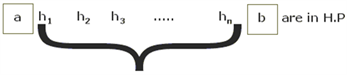# Harmonic Progression

The Harmonic Progression is a part of the progression topic from CAT quantitative aptitude section. Learn the detailed concepts of the topic with easily-understandable explanations and solved illustrations.

### Introduction: Harmonic Progression

Numbers a, b, c are said to be in Harmonic Progression HP, if the reciprocals of these numbers (1/a,1/b,1/c) are in an AP. Also, no term in an HP, can be zero.

Example: Take the numbers: 1, 1/3 and 1/5.

Now, the reciprocals of the given numbers are 1, 3, 5 which are in AP; so, it can be said that 1, 1/3, 1/5 are in HP.

### General form Of an HP

The nth term of an HP = 1/((nth term of corresponding AP))

Example: take the HP: 1/2, 1/5, 1/8

The corresponding AP is 2, 5, 8 with first term 2 and common difference = 3.

The 6th term in this AP = 2 + (5)(3) = 17.

The corresponding 6th term in the HP = 1/17.

#### Example Question:

If the first two terms in an HP are 24 and 12, then find the 4th term?

Solution:

Let 3rd term = x

Since the reciprocals are in AP, 1/24,1/12,1/x are in AP

i.e 1/x-1/12=1/12-1/24 1/x=1/6–1/24=1/8

Let the 4th term = y 1/y-1/8=1/8-1/12=>1/y=1/6=>y =6

Required answer, 4th term = 6

Alternately, if you take the percentage equivalents, it becomes easier as,

1/24= 4.16

1/12= 8.33. 4.16 & 8.33 are the first two terms in the AP,

3rd term = 12.5, 4th term = 16.66 = 1/6,

which implies that the 4th term in the HP = 6.

### Harmonic Mean (HM):

Let a and b be two given quantities. It is required to insert n harmonic means h1, h2, h3,….hbetween the quantities a and b=> 1/a,1/h1,1/h2,…..1/hn,1/b are in A.P

Harmonic mean of n numbers a1,a2,……an is given by: n/[1/a1 + 1/a2 + 1/a3 +?.1/an ]

#### Harmonic Mean Formula:

If a, b, c are in Harmonic Progression, then

HM, b =2/[1/a + 1/c ] = 2ac/((a + c) )

Harmonic mean of 4 numbers a, b, c, d = 4/((1/a + 1/b + 1/c +1/d) )

i.e. In an HP if you take three consecutive terms, middle term will be the harmonic mean of the first term and the third term.

### Application of Harmonic Mean

Harmonic mean helps to find out the average speed of a journey. The average speed when the distances covered are equal = HM of the speed.s

Let’s say a person P, covers a distance A to B at a speed of “a” kmph and a distance B to A at a speed of “b” kmph.

### Illustrations:

1. A person travels from A to B with a speed of 60 km/hr and returns from B to A at 40 km/hr. What is the average speed for the whole journey?

Solution:

Here since the distances covered are equal, average speed for the whole journey = Harmonic mean of the speeds =

HM of 60,40 = 2/[(1/60) + (1/40) ] = (2×60×40)/(60+40)= 48 km/hr.

2. Amit covers first 1/3rd of the distance walking at 2 km/hr, second 1/3rd of the distance running at 3km/hr and the rest cycling at 6 km/hr. Find the average speed for the whole journey?

a) 3 km/hr

b) 3.66 km/hr

c) 4 km/hr

d) 5 km/hr.

Solution:

Distances covered are equal for all 3 cases,

i.e. each time= 1/3rd of the total distance.

Hence, average speed = Harmonic mean of 2, 3 and 6

= 3/[(1/2)+ (1/3)+ (1/6) ] = 3 km/hr.

Stay tuned with Byju’s to learn more important topics of the CAT 2020 effectively from simple explanations and engaging video lessons. Also, get various practice questions sets, sample papers and previous year question papers to get acquainted with the type and difficulty of the CAT questions.